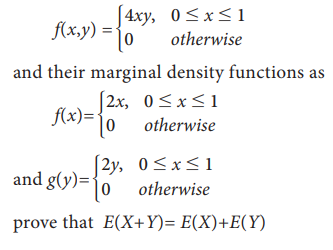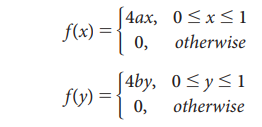Home | | Statistics 11th std | Addition and Multiplication Theorem on Expectations

# Addition and Multiplication Theorem on Expectations

Statistics : Random Variables and Mathematical Expectation

Addition and Multiplication Theorem on Expectations

## 1. Statement for Discrete random variable

If X and Y are two discrete random variables then

(X+Y) = E(X) + E(Y)

### Proof

Let the random variable X assumes the values x1, x2 ... xn with corresponding probabilities p1, p2…..pn, and the random variable y assume the values y1, y2 ……. ym with corresponding probabilities p1, p2…..pm## 2. Statement for Continuous random variable

Let X and Y are two continuous random variables with probability density functions f(x) and f(y) respectively. Then E (X + Y) = E(X) + E(Y)

Proof:### Remarks:

1. Statement: (aX+b) = aE(X)+b where a and b are constants.

Proof: E(aX+b) = E(aX)+E(b) by property 3

= aE(X)+b by property 2

(aX-b) = aE(X)-b### Example 9.23

Find the expectation of the sum of the number obtained on throwing two dice.

### Solution:

Let X&Y denote the number obtained on the I and II die respectively. Then each of them is a random variable which takes the value 1,2,3,4,5 and 6 with equal probability 1/6.Thus the expectation of the numbers obtained on two dices.

X+Y takes the values 2, 3…12 with their probability given by### Example 9.24

Let X and Y are two random variables with p.d.f given bySolution:## Discrete random variable:

Statement: If X and Y are two independent variables then E(XY) = E (X) E(Y)## Continuous random variable:

Statement: If X and Y are independent random variables Then E (XY) = E(X) E(Y)### Example 9.25

Two coins are tossed one by one. First throw is considered as X and second throw is considered as Y following joint probability distribution is given by,[getting Head is taken as 1 and Tail is taken as 0]

Verify E(XY)= E(X) E(Y)

### Solution:

A random variable XY can take the values 0 and 1[It is applicable only when X and Y are independent]

### Example 9.26

The independent random variables X and Y have the p.d.f given byProve that E(XY) = E(X) E(Y)

### Solution:

X and Y are independent

f(x,y) = f(x) × f(y)

f(x,y) = 4ax×4byStudy Material, Lecturing Notes, Assignment, Reference, Wiki description explanation, brief detail
11th Statistics : Chapter 9 : Random Variables and Mathematical Expectation : Addition and Multiplication Theorem on Expectations |